# A linear model with non-constant variances

## The package

The ‘lmvar’ package fits a Gaussian linear model. It differs from a classical linear model in that the variance is not constant. Instead, the variance has its own model, comparable to the model for the expected value. The classical linear model is provided by the function ‘lm’ in the package ‘stats’.

Working with the package is a lot like working with ‘lm’. A fit with ‘lmvar’ results in an ‘lmvar’ object, which is a list. Accessor functions are provided to extract the list members, such as the fitted coefficients $$\beta$$ and the log-likelihood. Various utility functions such as residuals to calculate residuals, AIC to calculate the AIC, fitted to obtain expected values, standard deviations and confidence intervals, etc., are also provided by the package.

The package is intended for people who run a classical linear model and want to see what happens if the restriction of a constant variance is dropped. Questions in this context are: does the allowance of heteroscedasticity result in a better fit, lower values for the AIC or BIC, smaller prediction errors, etc.?

## The model

The package fits the following model. A vector $$Y$$ of observations (also called ‘responses’) of length $$n$$ is a stochastic vector. It is distributed according to a multivariate Gaussian distribution:

$\begin{equation} Y \sim \mathcal{N}_n( \mu, \Sigma), \end{equation}$ where $$\mu$$ is the vector of expected values and $$\Sigma$$ the covariance matrix (also called the ‘variance-covariance matrix’). Just like in the standard linear model, the covariance matrix is taken to be a $$n \times n$$ diagonal matrix but contrary to the standard linear model, the diagonal entries need not be all the same: $\begin{equation} \Sigma_{ij} = \begin{cases} 0 & i \neq j\\ \sigma_i^2 & i=j \end{cases} \end{equation}$

### Model for the expectation values

As in the classical linear model, the vector of expectation values $$\mu$$ is given by $\begin{equation} \mu = X_\mu \beta_\mu \end{equation}$ where $$X_\mu$$ is the ‘model matrix’ or ‘design matrix’ for $$\mu$$ and $$\beta_\mu$$ the parameter vector for $$\mu$$. $$X_\mu$$ is a $$n \times k_\mu$$ matrix and $$\beta_\mu$$ a vector of length $$k_\mu$$.

### Model for the variances

Let $$\sigma$$ denotes the vector $$(\sigma_1, \dots, \sigma_n)$$. The model for $$\sigma$$ is $\begin{equation} \log \sigma = X_\sigma \beta_\sigma \end{equation}$ where $$\log \sigma$$ stands for the vector $$(\log\sigma_1, \dots, \log\sigma_n)$$, $$X_\sigma$$ is the ‘model matrix’ or ‘design matrix’ for $$\sigma$$ and $$\beta_\sigma$$ the parameter vector for $$\sigma$$. The logarithm is taken to be the ‘natural logarithm’ with base $$e$$. The dimensions of $$X_\sigma$$ are $$n \times k_\sigma$$ and $$\beta_\sigma$$ is a vector of length $$k_\sigma$$.

### Also know that…

The vector of observations $$Y$$ and the matrices $$X_\mu$$ and $$X_\sigma$$ are specified by the user. They must contain real values. The fit returns the maximum-likelihood estimators for $$\beta_\mu$$ and $$\beta_\sigma$$.

The model for both $$\mu$$ and $$\sigma$$ contains an intercept term by default. That means that the first column of both matrices is a column in which each matrix-element equals 1. The package will add this column to the user-suppplied matrices to ensure that the intercept term is present. There is no need for a user to include such a column in a user-supplied model-matrix. Intercept terms can be suppressed with the arguments intercept_mu = FALSE and intercept_sigma = FALSE of the function lmvar.

After adding the intercept columns (if not suppressed), the package will check whether the resulting matrices are full rank. If not, columns will be removed from each matrix until it is full rank.

The addition of an intercept column and, possibly, the removal of columns to obtain a full-rank matrix, imply that the actual matrices used in the fit can be different from the user-specified matrices. The matrices that are actually used in the fit are returned as members of the lmvar object.

Carrying out the fit boils down to solving a set of non-linear equations. By default this is carried out in the background by the function maxNR from the package ‘maxLik’ but it is possible to use another function from the same package.

More mathematical details about the model can be found in the vignette ‘Math’ which comes with this package. It can be viewed with vignette("Math") or vignette("Math", package="lmvar").

## Using the package

The main function in the package is lmvar. It carries out a fit and returns an lmvar object.

The user must specify a vector of observations and two model-matrices when calling lmvar. They must meet a number of conditions, which are described in detail in the on-line help for lmvar, to be viewed with the command ?lmvar. The most important conditions to keep in mind are:

• All observations and matrix elements must be real-valued.
• Missing values, values that are NaN etc., are not allowed.
• A matrix either has column names for all columns, or no column names at all. The same column name can appear for both $$X_\mu$$ and $$X_\sigma$$ but column names should be unique within each matrix. The intercept column that is added by lmvar (although one can suppress it), is called (Intercept) for $$X_\mu$$ and (Intercept_s) for $$X_\sigma$$.

With each column in $$X_\mu$$ corresponds an element in $$\beta_\mu$$. The name of that element is the corresponding column name. The same is true for $$X_\sigma$$ and $$\beta_\sigma$$.

It can happen that lmvar fails to solve the maximum-likelihood equations and exits with warnings. See here for the options you have in this case.

An lmvar object is a list whose members are intended to be extracted with the supplied accessor and utility functions. The only members for which no such functions have been implemented are:

• y: the user-supplied vector of observations
• X_mu: the actual, full-rank matrix $$X_\mu$$ including the intercept term (if not suppressed) that is used in the fit
• X_sigma: the actual, full-rank matrix $$X_\sigma$$ including the intercept term (if not suppressed) that is used in the fit
• slvr_log: log-output from the function maxNR. Only added to the lmvar object when requested.

Once lmvar has run and an lmvar-object has been created, one can obtain $$\beta_\mu$$ and $$\beta_\sigma$$ with the function coef. The function fitted allows one to obtain $$\mu$$ and $$\sigma$$. We refer to the package documentation (in particular the package index which can be viewed with help(package = "lmvar")) for a list of all available functions and function details.

## Demonstration

We demonstrate the package with the help of the dataframe cats which can be found in the MASS package.

# As example we use the dataset 'cats' from the library 'MASS'.
require(lmvar); require(MASS)

# A plot of the heart weight versus the body weight in the data set
plot(cats$Bwt, cats$Hwt, xlab = "Body weight", ylab = "Heart weight")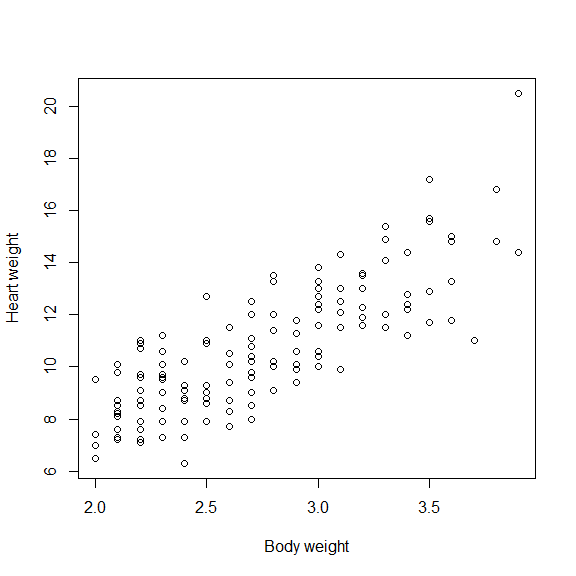We want to regress the cats heart weight ‘Hwt’ onto the body weight ‘Bwt’.

# Create the model matrix. It only contains the body weight. An intercept term will be added by 'lmvar'.
X = model.matrix(~ Bwt - 1, cats)

# Carry out the fit with the same model matrix for mu (the expected heart weight) and for log sigma (the standard deviation)
fit = lmvar(cats$Hwt, X_mu = X, X_sigma = X) We have now created the object fit, which is our object of class lmvar. To obtain a first impression of the fit, we look at the summary summary(fit) ## Call: ## lmvar(y = cats$Hwt, X_mu = X, X_sigma = X)
##
## Number of observations:  144
## Degrees of freedom    :  4
##
## Z-scores:
##     Min      1Q  Median      3Q     Max
## -2.4159 -0.7261 -0.0655  0.6703  2.5998
##
## Coefficients:
##                Estimate Std. Error z value  Pr(>|z|)
## (Intercept)   -0.012179   0.679820 -0.0179  0.985707
## Bwt            3.904310   0.258964 15.0766 < 2.2e-16 ***
## (Intercept_s) -0.522274   0.337044 -1.5496  0.121244
## Bwt_s          0.315837   0.121843  2.5922  0.009537 **
## ---
## Signif. codes:  0 '***' 0.001 '**' 0.01 '*' 0.05 '.' 0.1 ' ' 1
##
## Standard deviations:
##    Min     1Q Median     3Q    Max
## 1.1156 1.2265 1.3916 1.5422 2.0330
##
## Comparison to model with constant variance (i.e. classical linear model)
## Log likelihood-ratio: 4.069774
## Additional degrees of freedom: 1
## p-value for difference in deviance: 0.00433

The first line shows the call that created fit. Then, we are told something about the distribution of the standard scores (also called the z-scores).

Next, the summary shows the matrix with the coefficients $$\beta_\mu$$ and $$\beta_\sigma$$. The coefficients $$\beta_\mu$$ are (Intercept), and Bwt. The coefficients $$\beta_\sigma$$ are (Intercept_s), and Bwt_s. They are called this way to distinguish them from the coefficients for $$\beta_\mu$$. In cases where there is no risk of confusion, the true names of the coefficients $$\beta_\sigma$$ will be used, which are (Intercept_s) and Bwt.

The matrix with coefficients shows that Bwt and Bwt_s are statistically significant at the 5% level, but the intercept terms are not.

The next piece of information gives an impression of the distribution of the standard deviations $$\sigma$$.

Finally the model is compared to a classical linear model with the same model matrix $$X_\mu$$ but a standard deviation that is the same for all observations. The summary shows the difference in log-likelihood between the two models and the difference in degrees of freedom. Twice the difference in log-likelihood is the difference in deviance, for which a p-value is calculated. The p-value of 0.00433, indicates that the lmvar fit is a better fit than the classical linear model at the 5% confidence level. I.e., it makes sense to let the standard deviation vary instead of keeping it fixed.

Another useful, high-level check of the fit is to look at a number of diagnostic plots with the command plot (fit).

The number of observations in the fit and the log-likelihood are

nobs(fit)
##  144
logLik(fit)
## 'log Lik.' -252.991 (df=4)

The rank of the matrix $$X_\mu$$ used in the fit is

dfree(fit, sigma = FALSE)
##  2

The value 2 is correct: the user-supplied matrix had 1 column and lmvar added an intercept column. The two columns are linearly independent so no column had to be removed.

We run the regression again, but this time without intercept terms

fit = lmvar(cats$Hwt, X_mu = X, X_sigma = X, intercept_mu = FALSE, intercept_sigma = FALSE) The rank of $$X_\mu$$ as used in the fit is now equal to 1: dfree(fit, sigma = FALSE) ##  1 The summary no longer shows the intercept terms: summary(fit) ## Call: ## lmvar(y = cats$Hwt, X_mu = X, X_sigma = X, intercept_mu = FALSE,
##     intercept_sigma = FALSE)
##
## Number of observations:  144
## Degrees of freedom    :  2
##
## Z-scores:
##     Min      1Q  Median      3Q     Max
## -2.2212 -0.6966 -0.0686  0.7168  3.1238
##
## Coefficients:
##       Estimate Std. Error z value  Pr(>|z|)
## Bwt   3.903044   0.044278 88.1489 < 2.2e-16 ***
## Bwt_s 0.134489   0.021302  6.3135 2.728e-10 ***
## ---
## Signif. codes:  0 '***' 0.001 '**' 0.01 '*' 0.05 '.' 0.1 ' ' 1
##
## Standard deviations:
##    Min     1Q Median     3Q    Max
## 1.3086 1.3625 1.4378 1.5021 1.6896

The summary overview has left out the comparison with the classical linear model as well. The classical linear model and the model in fit are no longer nested models because of the absence on an intercept term in $$X_\sigma$$.

Let’s see whether the z-scores appear to be correlated with the body weight

sigma = fitted(fit, mu = FALSE)
plot(cats$Bwt, residuals(fit) / sigma, xlab = "Body weight", ylab = "z-score") abline(0, 0, col = "red")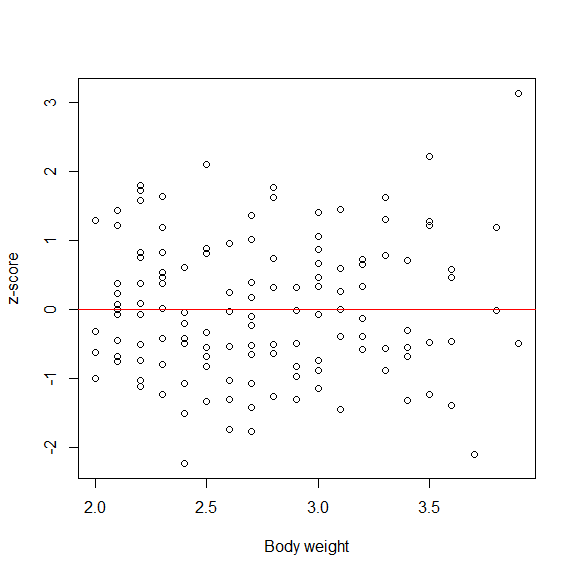Let’s also plot the average heart weights versus the body weight, with a 95% confidence interval as error bar. mu = fitted(fit, sigma = FALSE) plot(cats$Bwt, mu, xlab = "Body weight", ylab = "Average heart weight", ylim = c(7, 16))

intervals = fitted(fit, interval = "confidence", level = 0.95)
lwr = intervals[, "mu_lwr"]
upr = intervals[, "mu_upr"]

segments(cats$Bwt, lwr, cats$Bwt, upr)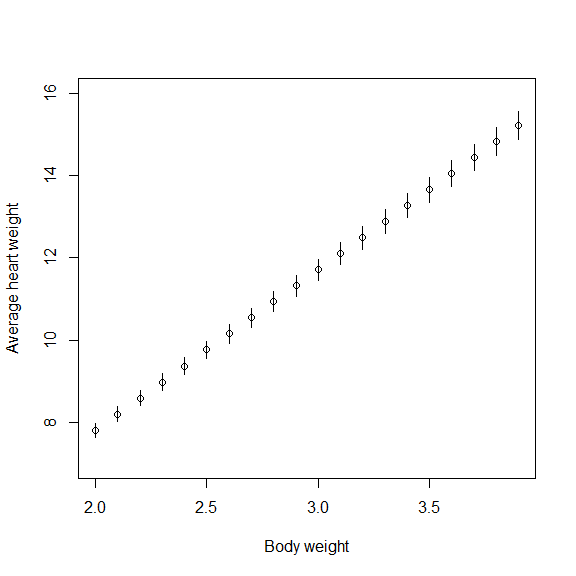We carry out a classical linear fit, without an intercept term in the model matrix

fit_lm = lm(cats$Hwt ~ Bwt - 1, cats) and we compare AIC and BIC values AIC(fit); AIC(fit_lm) ##  512.7867 ##  518.3905 BIC(fit); BIC(fit_lm) ##  518.7263 ##  524.3301 Both AIC and BIC favor the fit with lmvar over the fit with lm. We plot again the expected heart weight versus the body weight, but now we add the predictions of the classical linear fit as red points plot(cats$Bwt, mu, xlab = "Body weight", ylab = "Average heart weight", ylim = c(7, 16))
segments(cats$Bwt, lwr, cats$Bwt, upr)
points(cats$Bwt, fitted(fit_lm), col = "red")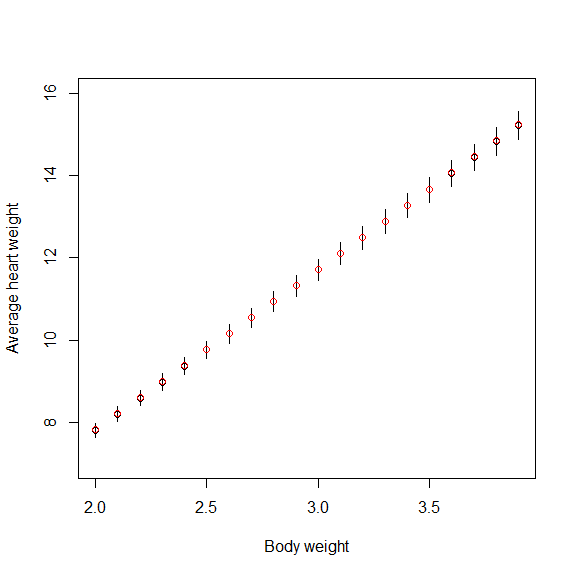Te plot shows that the predicted values from the classical linear model are nearly the same as the predictions from lmvar. We can also plot the fitted standard deviations with their 95% confidence interval: plot(cats$Bwt, sigma, xlab = "Body weight", ylab = "St dev of heart weight", ylim = c(1, 2))

lwr = intervals[, "sigma_lwr"]
upr = intervals[, "sigma_upr"]
segments(cats$Bwt, lwr, cats$Bwt, upr)

sigma_lm = summary(fit_lm)$sigma abline(sigma_lm, 0, col = "red")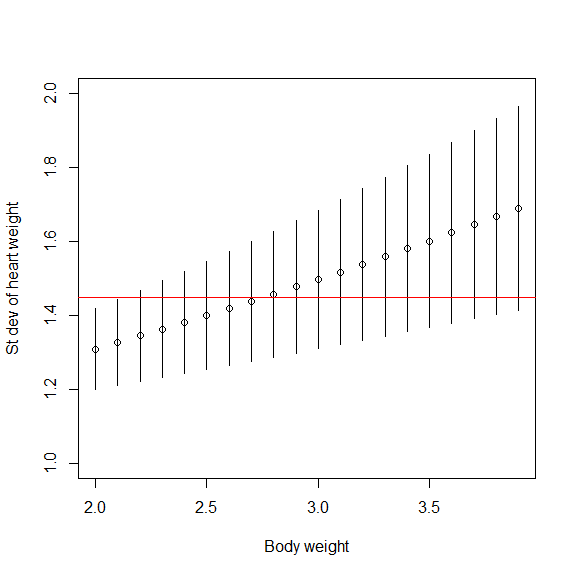The red line is the standard deviation that is returned from the classical linear model. The coefficients $$\beta_\mu$$ and $$\beta_\sigma$$ that were displayed in the summary-overview, are obtained by coef(fit) ## Bwt Bwt_s ## 3.9030442 0.1344892 The suffix ’_s’ is added to show that ‘Bwt_s’ is the coefficient $$\beta_\sigma$$. If we ask for $$\beta_\sigma$$ only, the real name of the coefficient is used coef(fit, mu = FALSE) ## Bwt ## 0.1344892 We conclude this demonstration with the covariance matrix for the combined coefficients $$(\beta_\mu, \beta_\sigma)$$ vcov(fit) ## Bwt Bwt_s ## Bwt 0.00196053 0.0000000000 ## Bwt_s 0.00000000 0.0004537699 Hopefully this demonstration has given an idea of how to work with the package. The documentation of the individual functions contains further examples. ## Functions in the package We refer to the package index for a list of all available functions. The index can be viewed with help(package="lmvar"). Various generic functions from the ‘stats’ package work for an ‘lmvar’ object as well. Examples are BIC and confint. ### Plots The function plot.lmvar returns a number of diagnostic plots for an ‘lmvar’ object, similar to the function plot.lm for an ‘lm’ object. The function plot_qq produces a QQ-plot for one or two fits. They can be of class ‘lm’ or class ‘lmvar’. It is also possible that one id of class ‘lm’ and the other of class ‘lmvar’. The function plot_qdis produces a plot of the distribution of quantiles. Like plot_qq, one can specify two plots, each of class ‘lm’ or class ‘lmvar’. ### Variable selection The package provides the function fwbw for a selection of the independent model-variables (also called the ‘predictors’ or ‘covariates’). It searches for an optimal subset of variables by means of a forward / backward-stepping algorithm. The function works on a ‘lmvar’ object but also on a ‘lm’ object. See the function documentation of fwbw for details. ### Cross-validation The package provides the functions cv.lm and cv.lmvar for cross-validation of prediction errors or any other function of choice. The former function works on an ‘lm’ object, the latter on a ‘lmvar’ object. See the function documentation for details. ## The class ‘lmvar_no_fit’ The package ‘lmvar’is concerned mostly with objects of class ’lmvar’. However, it also contains the function lmvar_no_fit which creates an object of class ‘lmvar_no_fit’. This class is like the class ‘lmvar’ but misses any information that is the result of a model fit. The class ‘lmvar’ is an extension of the class ‘lmvar_no_fit’. This means that whenever an object of class ‘lmvar_no_fit’ is required, an object of class ‘lmvar’ can be used as well. An example is the function nobs.lmvar_no_fit supplied by this package. It takes as input an object of class ‘lmvar_no_fit’. Therefore it will also accept an object of class ‘lmvar’. ## What if the fit does not converge? It can happen that the function lmvar exits with a warning that it had trouble carrying out the model fit. The warning will be like ‘Last step could not find a value above the current’ or ‘Log-likelihood appears not to be at a maximum!’. This is means that the iterative fitting procedure did not converge in a satisfactory manner. In our experience, this is most likely to happen when the model for $$\sigma$$ has many degrees of freedom, i.e., the model matrix $$X_\sigma$$ has many columns. What are your options if this happens? You can try the following strategies. • Check whether the model for $$\sigma$$ contains factor levels which occur only a few times, or which occur almost always. Such levels are columns in $$X_\sigma$$ where nearly all elements are equal to 0 and a few equal to 1, or nearly all elements are equal to 1 and a few equal to zero. Remove these columns from $$X_\sigma$$ and run lmvar again. • Run lmvar with the argument control = list(remove_df_sigma_post = TRUE). With this option, lmvar tries to remove degrees of freedom from the model for $$\sigma$$ that prevent the fit to converge. See the vignette ‘Math’ for a mathematical background of this option. • Create the model by starting with a model with only 2 degrees of freedom and gradually adding degrees of freedom, avoiding ones that prevent the fit to converge. This can be done by first running lmvar_no_fit (with the same arguments as lmvar). The resulting object is then input for the function fwbw. This function must be run with argument fw = TRUE. The function fwbw requires one to select a function that measures the goodness of fit. Reasonable choices for this function are e.g. AIC or BIC. The output of fwbw contains a model-fit which is hopefully one you can work with. ## Other packages There are several other package that can fit the same model as ‘lmvar’. Below, we mention the ones we are aware of. The ‘lmvar’ package has been developed as a relatively simple next step for users who typically run a linear regression, but want to gain experience with a heterscedastic model. Depending on one’s taste and needs, one might need or prefer another package though. If we can, we demonstrate the alternatives with the same example we have used to demonstrate ‘lmvar’. ### The function ‘remlscore’ The function remlscore from the package ‘statmod’ (Giner and Smyth 2016) fits our example as follows. require(statmod) # Run the regression including intercept terms intercept = rep(1, nrow(cats)) fit = remlscore( cats$Hwt, cbind(intercept, X), cbind(intercept, X))

The coefficients $$\beta$$ for the expected value can be obtained as fit$beta. The coefficients for the logarithm of the standard deviation as fit$gamma. By definition, the latter coefficients are twice the value calculated by lmvar.

### The function ‘crch’

The function crch from the package ‘crch’ (Messner, Mayr, and Zeileis 2016) fits our example as follows.

require(crch)

# Run the regression including intercept terms
fit = crch(Hwt ~ Bwt | Bwt , data = cats)

All coefficients $$\beta$$ can be obtained with coef(fit).

### The function ‘gam’

The function gam from the package ‘mgcv’ (Wood 2011) fits our example as follows.

require(mgcv)

# Run the regression including intercept terms
fit = gam(list(Hwt ~ Bwt, ~ Bwt) , data = cats, family = gaulss())

All coefficients $$\beta$$ can be obtained with coef(fit).

### The function ‘gamlss’

The function gamlss from the package ‘gamlss’ (Rigby and Stasinopoulos 2005) fits our example as follows.

require(gamlss)

# Run the regression including intercept terms
fit = gamlss(Hwt ~ Bwt, ~ Bwt, data = cats)

The coefficients $$\beta$$ for the expected value can be obtained as coef(fit). The coefficients for the log of the standard deviation are obtained by coef(fit, what = "sigma").

### Other options

Other functions that allow for a model of the dispersion are, e.g., hglm in the package hglm (Ronnegard, Shen, and Alam 2010) and geese in the package geepack (Hojsgaard, Halekoh, and Yan 2006). These models are more complicated though, and require a level of expertise not required by lmvar.

## Acknowledgements

We thank Prof. Dr. Eric Cator for his valuable comments and suggestions. Prof. Dr. Achim Zeileis brought the packages ‘crch, ’mgcv’ and ‘gamlss’ to our attention.

This package uses the package maxLik (Henningsen and Toomet 2011) to find the maximum likelihood. The package matrixcalc is used to check properties of the Hessian. The package Matrix is used to support matrices of class ‘Matrix’.